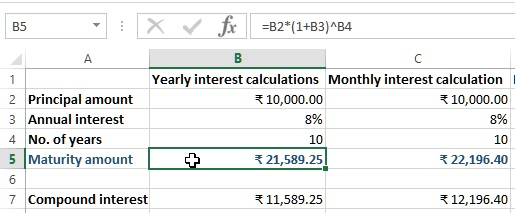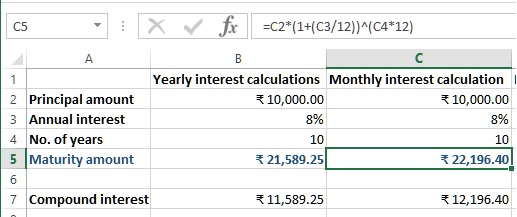# Compound interest calculations in Excel

##### How to calculate compound interest in Excel?

In the previous post, we learnt 'What if I had invested calculations in Excel'. In this post, we shall learn how to calculate compound interest in MS Excel.

Excel doesn't have an inbuilt function to calculate compound interest. So we will do some manipulations to calculate compound interest.

In below example, we have '=B2*(1+B3)^B4' formula in B5 cell.

• B2 is Principal amount to pay every year
• B3 is annual interest rate
• B4 is payment term in year
This will give us the compounded maturity amount calculated yearly.To calculate the compounded maturity amount monthly, we need '=C2*(1+(C3/12))^(C4*12)' formula, where

• we have divided interest rate by 12 and
• multiplied number of years by 12

(as 12 months in a year) and the result is below.If you see both maturity amounts, there is slight difference because of yearly and montly calculation of compound interest.

Views: 4437 | Post Order: 35